Function Repository Resource:

# MeanMedianLogNormalDistribution

Create a lognormal distribution using mean and median as parameters instead of the conventional parameters

Contributed by: Seth J. Chandler
 ResourceFunction["MeanMedianLogNormalDistribution"][mean,median] creates a LogNormalDistribution whose mean is mean and whose median is median.

## Details and Options

The median must be less than or equal to the mean.

## Examples

### Basic Examples (2)

Create a LogNormalDistribution whose mean is 3 and whose median is ⅇ:

 In:=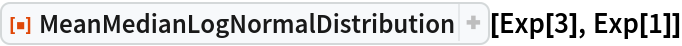Out=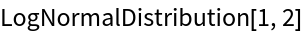Create a lognormal distribution whose mean is 7000 and whose median is 2000:

 In:=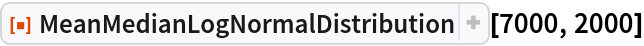Out=### Scope (1)

The function handles symbolic parameters:

 In:=Out=### Applications (1)

Show how decreasing the median of a lognormal distribution affects the associated PDF:

 In:=Out=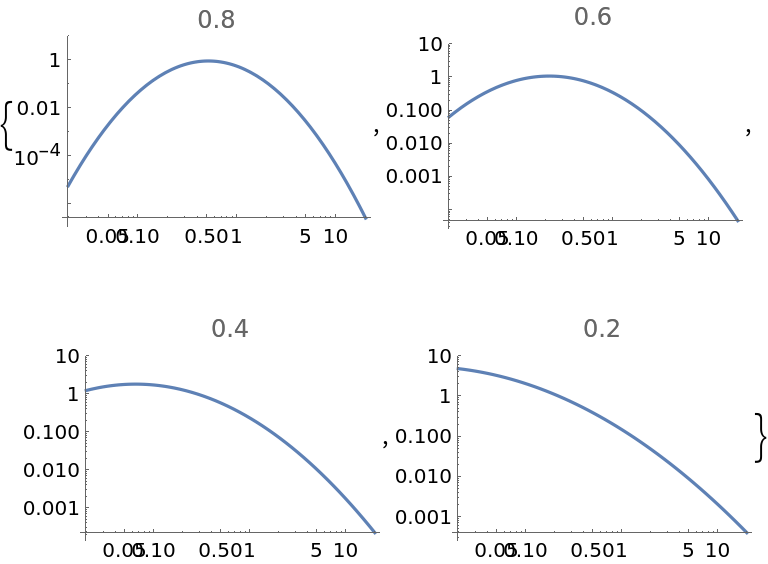### Properties and Relations (2)

An alternative parameterization would use the mean and the ratio of the median to the mean:

 In:=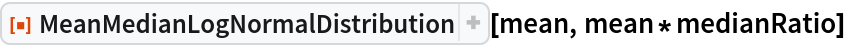Out=For other distributions, one can perform similar reparameterizations by using Solve, Reduce or similar methods:

 In:=Out=### Possible Issues (1)

If the median is greater than the mean, one gets a LogNormalDistribution with impermissible imaginary components:

 In:=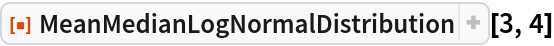Out=### Neat Examples (2)

Observations of health claims show that the mean is 7000 and the median is 2500. Compute the fraction of total claims incurred by persons in the top 1% of claims:

 In:=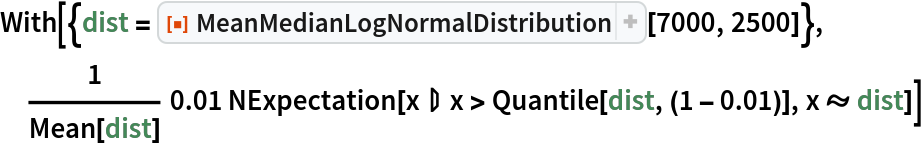Out=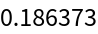Make a table showing the corresponding fraction as one examines the top q percent of claims:

 In:=Out=Seth J. Chandler

## Version History

• 1.0.0 – 23 August 2019